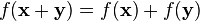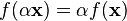# Linear mapping facts for kids

Kids Encyclopedia Facts

In mathematics, a linear mapping (or linear transformation) is a mapping f between vector spaces that preserves addition and scalar multiplication.

## Definition

Let V and W be vector spaces over the same field K. A function f: VW is said to be a linear mapping if for any two vectors x and y in V and any scalar α in K, the following two conditions are satisfied:$f(\mathbf{x}+\mathbf{y}) = f(\mathbf{x})+f(\mathbf{y}) \!$$f(\alpha \mathbf{x}) = \alpha f(\mathbf{x}) \!$

Sometimes a linear mapping is called a linear function. However in basic mathematics, a linear function means a function whose graph is a line.

## Related pagesLinear mapping Facts for Kids. Kiddle Encyclopedia.